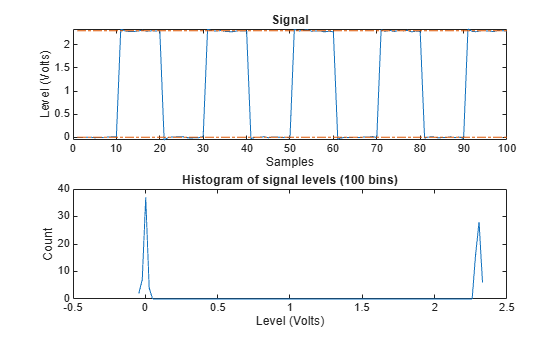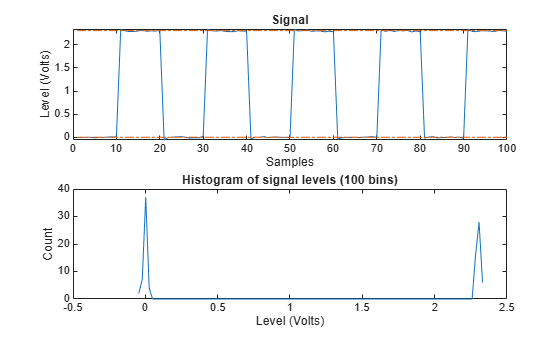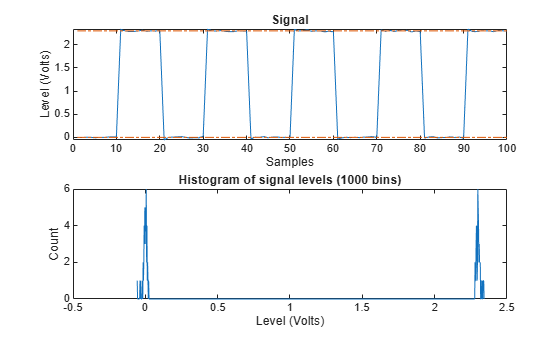# statelevels

State-level estimation for bilevel waveform with histogram method

## Syntax

levels = statelevels(x)
levels = statelevels(x,nbins)
levels = statelevels(x,nbins,method)
levels = statelevels(x,nbins,method,bounds)
[levels,histogram] = statelevels(___)
[levels,histogram,binlevels] = statelevels(___)
statelevels(___)

## Description

example

levels = statelevels(x) estimates the low and high state levels in the bilevel waveform x using the histogram method. For more information, see Algorithms.

example

levels = statelevels(x,nbins) specifies the number of bins to use in the histogram as a positive scalar.

example

levels = statelevels(x,nbins,method) estimates state levels using the mean or mode of the subhistograms.
levels = statelevels(x,nbins,method,bounds) specifies the lower and upper bounds of the histogram in the two-element real row vector bounds. statelevels ignores any values of x that lie outside these bounds when it computes the histogram.
[levels,histogram] = statelevels(___) returns the histogram of the values in x.
[levels,histogram,binlevels] = statelevels(___) returns the centers of the histogram bins.

example

statelevels(___) displays a plot of the signal and the corresponding histogram.

## Examples

collapse all

Estimate the low- and high-state levels of 2.3 V underdamped clock data. Plot the data with the estimated state levels and subhistograms.

load('clockex.mat','x') statelevels(x)ans = 1×2 0.0027 2.3068 

Estimate the low- and high-state levels of 2.3 V underdamped clock data sampled at 4 MHz.

Use the default number of bins and modes of the subhistograms to estimate the state levels.

load('clockex.mat','x','t') levs = statelevels(x)
levs = 1×2 0.0027 2.3068 

Plot the clock data with the lines indicating the estimated low- and high-state levels.

statelevels(x)ans = 1×2 0.0027 2.3068 

Estimate the low- and high-state levels of 2.3 V underdamped clock data sampled at 4 MHz.

Use the default number of bins and means of the subhistograms to estimate the state levels. Plot the clock data with the lines indicating the estimated low- and high-state levels.

load('clockex.mat','x','t') statelevels(x,1e3,'mean')ans = 1×2 -0.0014 2.3014 

Estimate the low- and high-state levels of 2.3 V underdamped clock data sampled at 4 MHz. Return the histogram counts and histogram bin centers used in the histogram method. Use four bins.

load('clockex.mat','x','t') [levs,histog,bilevs] = statelevels(x,4)
levs = 1×2 0.2427 2.0428 
histog = 4×1 50 0 0 50 
bilevs = 4×1 0.2427 0.8427 1.4428 2.0428 

Plot the waveform and annotate the levels.

statelevels(x,4)ans = 1×2 0.2427 2.0428 

## Input Arguments

collapse all

Bilevel waveform, specified as a real-valued vector.

Number of histogram bins, specified as a real positive scalar.

State-level estimation method in the subhistograms, specified as 'mode' or 'mean'. method specifies the statistic to use for the estimation of the low- and high-state levels. See Algorithms.

Histogram lower and upper bounds, specified as a two-element real row vector. statelevels ignores any values of x that lie outside these bounds when it computes the histogram.

## Output Arguments

collapse all

Levels of low and high states, returned as a two-element positive row vector. The vector of state levels is estimated by the histogram method. The first element of levels is the low-state level and the second element is the high-state level.

Histogram counts, returned as a column vector with nbins elements containing the number of data values in each histogram bin.

Histogram bin centers, returned as a column vector. The column vectors contain the bin centers for the histogram counts in histogram

collapse all

### State

A state is a particular level, which can be associated with an upper- and lower-state boundary. States are ordered from the most negative to the most positive. In a bilevel waveform, the most negative state is the low state. The most positive state is the high state.

### State-Level Tolerances

You can specify lower- and upper-state boundaries for each state level. Define the boundaries as the state level plus or minus a scalar multiple of the difference between the high state and the low state. To provide a useful tolerance region, specify the scalar as a small number such as 2/100 or 3/100. In general, theregion for the low state is defined aswhereis the low-state level andis the high-state level. Replace the first term in the equation withto obtain thetolerance region for the high state.

This figure shows lower and upper 5% state boundaries (tolerance regions) for a positive-polarity bilevel waveform. The thick dashed lines indicate the estimated state levels.## Algorithms

statelevels uses the histogram method to estimate the states of a bilevel waveform. The histogram method is described in . The steps of this method are:

1. Determine the maximum and minimum amplitudes and amplitude range of the data.

2. For the specified number of histogram bins, determine the bin width, which is the ratio of the amplitude range to the number of bins.

3. Sort the data values into the histogram bins.

4. Identify the lowest-indexed histogram bin, ${i}_{\text{low}}$, and highest-indexed histogram bin, ihigh, with nonzero counts.

5. Divide the histogram into two subhistograms:

The indices of the lower histogram bins are ${i}_{\text{low}}\le i\le \frac{1}{2}\left({i}_{\text{high}}-{i}_{\text{low}}\right)$.

The indices of the upper histogram bins are ${i}_{\text{low}}+\frac{1}{2}\left({i}_{\text{high}}-{i}_{\text{low}}\right)\le i\le {i}_{\text{high}}$.

6. Compute the state levels by determining the mode or mean of the lower and upper histograms.

 IEEE® Standard on Transitions, Pulses, and Related Waveforms, IEEE Standard 181, 2003, pp. 15–17.